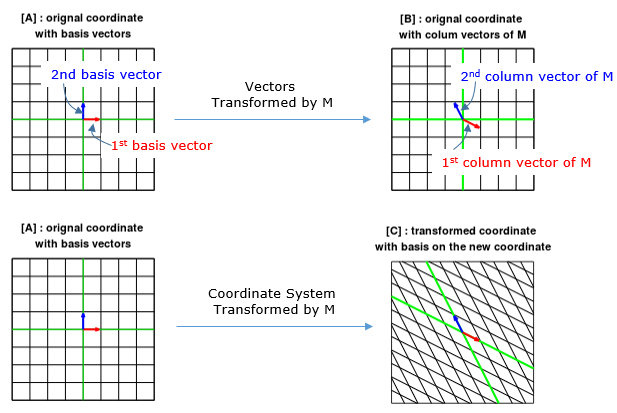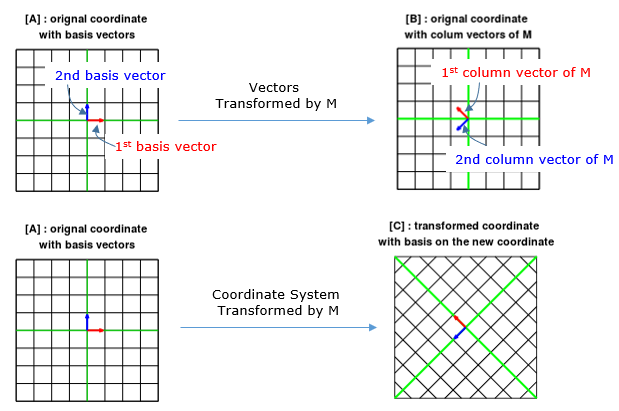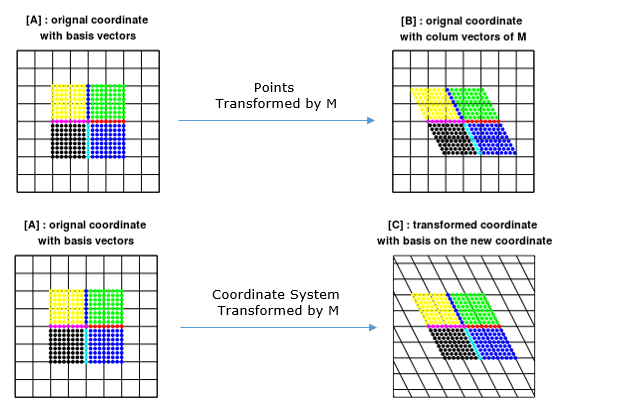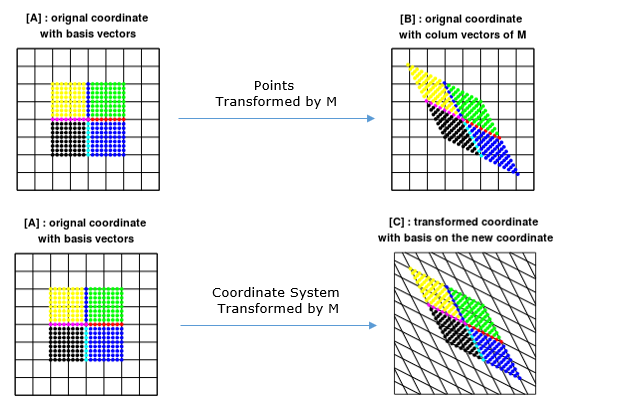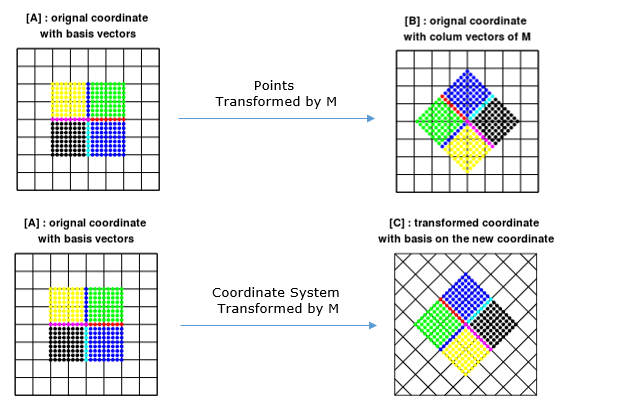Engineering Math - Quick Reference                                 Home : www.sharetechnote.com Matrix- Intuitive Property   My own image for a matrix is a kind of machine that is doing things as follows. As you see in the illustration, Matrix is taking in a shape (geometrical shape/object) and transform (change shape) them in mainly three different way as follows. i) Scale (magnify or shrink) ii) Rotate iii) Skew In reality, a matrix can do more than one type of transformation like "Scale and Skew", "Scale and Rotate and Skew" etc. I will talk about this kind of transformation in this section and I hope you can have some intuitive understanding of the propery of a matrix since it can be visualized as in this section.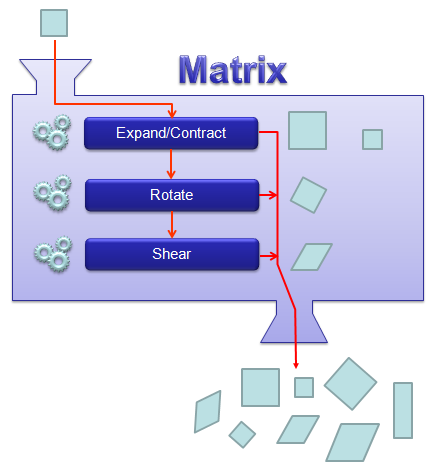I will write this note at two different angles.       Matrix as a Shape Transformer   I think one of the best way to understand the characteristics of a Matrix is to apply it for an shape in a graphical coordinates and observe the result. Of course, there would be a certain limitation in this method since we can only visualize three dimensional shape and as a result the dimension of the matrix we can visualize would be 3 x 3 (or 4 x 4 in some cases). But if you build up a solid intuitive understanding of a properties of a matrix in this way, you can easily extend the understanding to any size of the matrix and, more importantly, you can understand more easily a mathematical model represented in the matrix format.   I will use a shape in two dimensional coordinate and 2 x 2 matrix applying to the shape on the coordinate. Here you see coordinates labeled (x1,y1) and (x2,y2). (x1,y1) represents each points before it is transformed by the matrix and (x2,y2) is the new points after (x1,y1) is transformed by the matrix.   Let's look at the first case. The first matrix is what we call Identity Matrix which has the value '1' in all the elements on diagonal line running from left top to right bottom and all the other elements are set to be '0'. What is the result of the transformation of a shape transformed by the Identity Matrix ? The answer is "No change".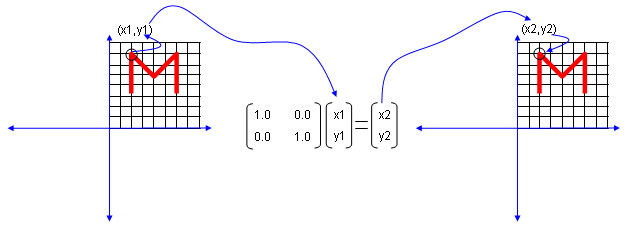Next look at another matrix shown below. This matrix also looks similar to diagonal matrix but not exactly same. The difference is that the first element on the diagonal line is '-1' in stead of '1'.  What is the result ? The shape is flipped around y axis.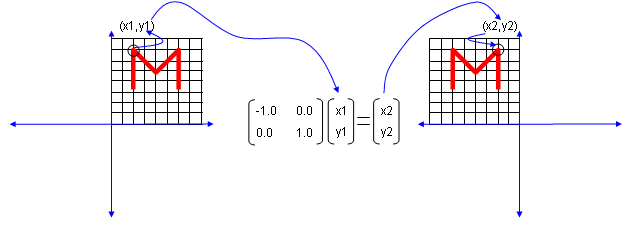Next look at another matrix shown below. This matrix also looks similar to diagonal matrix but not exactly same. The difference is that the second element on the diagonal line is '-1' in stead of '1'.  What is the result ? The shape is flipped around x axis.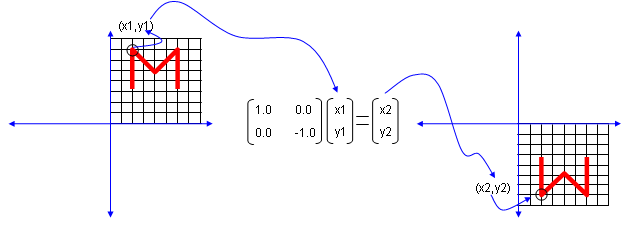Next look at another matrix shown below. In this case, all the elements on the diagonal lines is set to be '-1' instead of '0'.  What is the result ? It became reflected around the point (0,0). You can interpret this in two steps. At the first step, the shape is flipped around y axis. and at the second step the shape is fliped around x axis.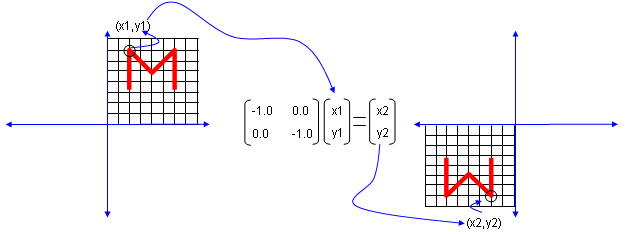Now let's look at another matrix as shown below. This time you see all '0's on the diagonal line and now you see a non-zero value out side of diagonal line. What is the result ? The image shears.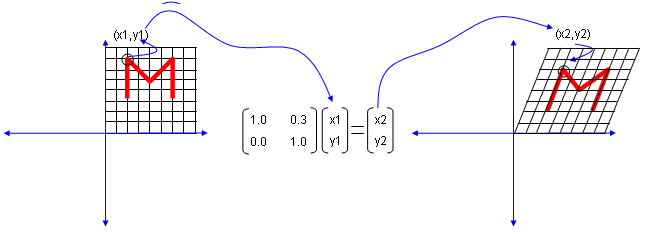Now let's look at another matrix as shown below. This time you see the non-zero value in all the elements. This is tricky to analyze since these matrix can do almost everything described above.. but if the numbers in the elements can be represented as trigonometrix functions in the following format. This matrix can rotate the image as shown below.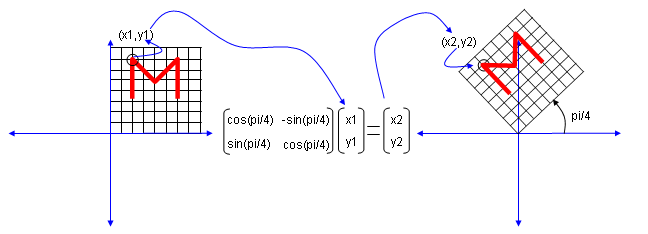Actually this is only a few of the examples.. you can try any numbers in the matrix and apply to some shape and try to correlate those numbers to the result of the transformation until you build up your own intuition of figuring out the characteristics of a matrix.       Matrix as a Coordinate System Transformer   Now I will introduce you another way to interpret the meaning of a matrix in terms of transformation. If a matrix is given in a well known form (well known numbers) as shown in previous section, you may figure out the characteristics of the matrix right away. But you may find it difficult to figure out the meaning of the matrix if the numbers in the matrix is not so familiar to you.  What I am going to introduce in this section would help you understand the meaning of the matrix in any form of the matrix.   Let me explain with a specific example. Let's assume that we have a matrix as shown below.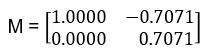First, let's think of this matrix as a combination of two column vectors and represent the two column vectors in a coordinate system. I will show you two different way of representation of the column vectors as shown below.   In Interpretation (I), you have the two column vectors plotted as in the plot [B]. In this interpretation, you can say two basis vector shown in plot [A] is transformed to different coordinates (vectors) in Plot [B] which is represented by the column vectors of the matrix M. More specifically, the vector (0,1) is transformed to coordinate (-0.7071,0.7071) by the matrix M and the vector (1,0) is transformed to coordinate (1.0,0.0) by the matrix M.   In Interpretation (II), you see the same column vectors in plot [C]. But you would notice that the coordinates of the vectors (meaning the numbers in the vectors) are different from the one in plot [B]. The coordinate in plot [C] is same as in plot [A]. But you see the obvious differences of the two sets of vectors in the plot [A] and plot [C]. How can the different looking vectors can have the same coordinate (coordinate value) ? If you look at the plots a little bit more carefully, you would notice that the coordinate system (grid lines) in plot [C] is different from plot [A]. In this case, we can interpret that the matrix transforms the whole coordinate system and each individual coordinate (the coordinate of individual vectors) stays same in the new (transformed) coordinate. In this specific example, you can say the vector (0,1) in original coordinate system (plot [A])  is mapped to the same coordinate (0,1) in the new (transformed) coordinate in plot [C] and the vector (1,0) in original coordinate system (plot [A])  is mapped to the same coordinate (1,0) in the new (transformed) coordinate in plot [C]   NOTE : Click here or on the image, it will lead you to slide show pages and you would get more intuitive understandings.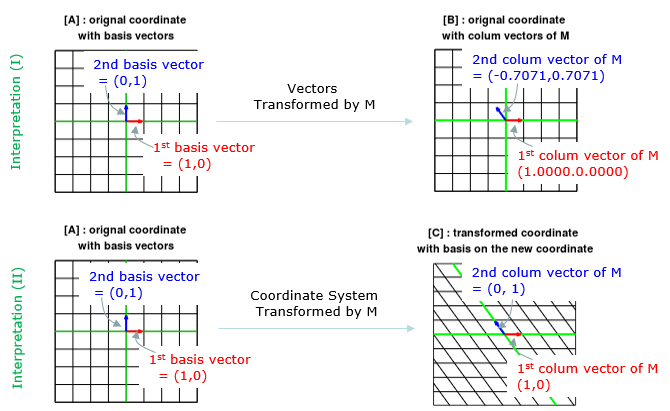For more intutive understandings, I put some more examples as shown below. Click on the images for the full slideshow pages.